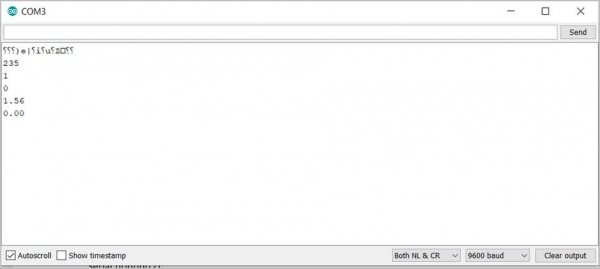# Convert string to integer/ float in Arduino

In order to convert a string to an integer or a float, the .toInt() and .toFloat() functions can be used. Of course, the string should actually correspond to the integer or floating-point value. For instance, "1.87" can be converted to float. But it doesn't make sense to convert "Hello" to float. The below example code illustrates the conversions −

## Example

void setup() {
Serial.begin(9600);
Serial.println();
// put your setup code here, to run once:
String s1 = "235";
String s2 = "1.56";
String s3 = "Hello";
int i1 = s1.toInt();
int i2 = s2.toInt();
int i3 = s3.toInt();
float f1 = s2.toFloat();
float f2 = s3.toFloat();
Serial.println(i1);
Serial.println(i2);
Serial.println(i3);
Serial.println(f1);
Serial.println(f2);
}
void loop() {
// put your main code here, to run repeatedly:
}

The Serial Monitor output is shown below −

## OutputAs you can see, the integer and float conversions for "Hello" are 0. The conversion of floating point to integer happens through chopping, as can be seen. The integer value of 1.56 was printed as 1.